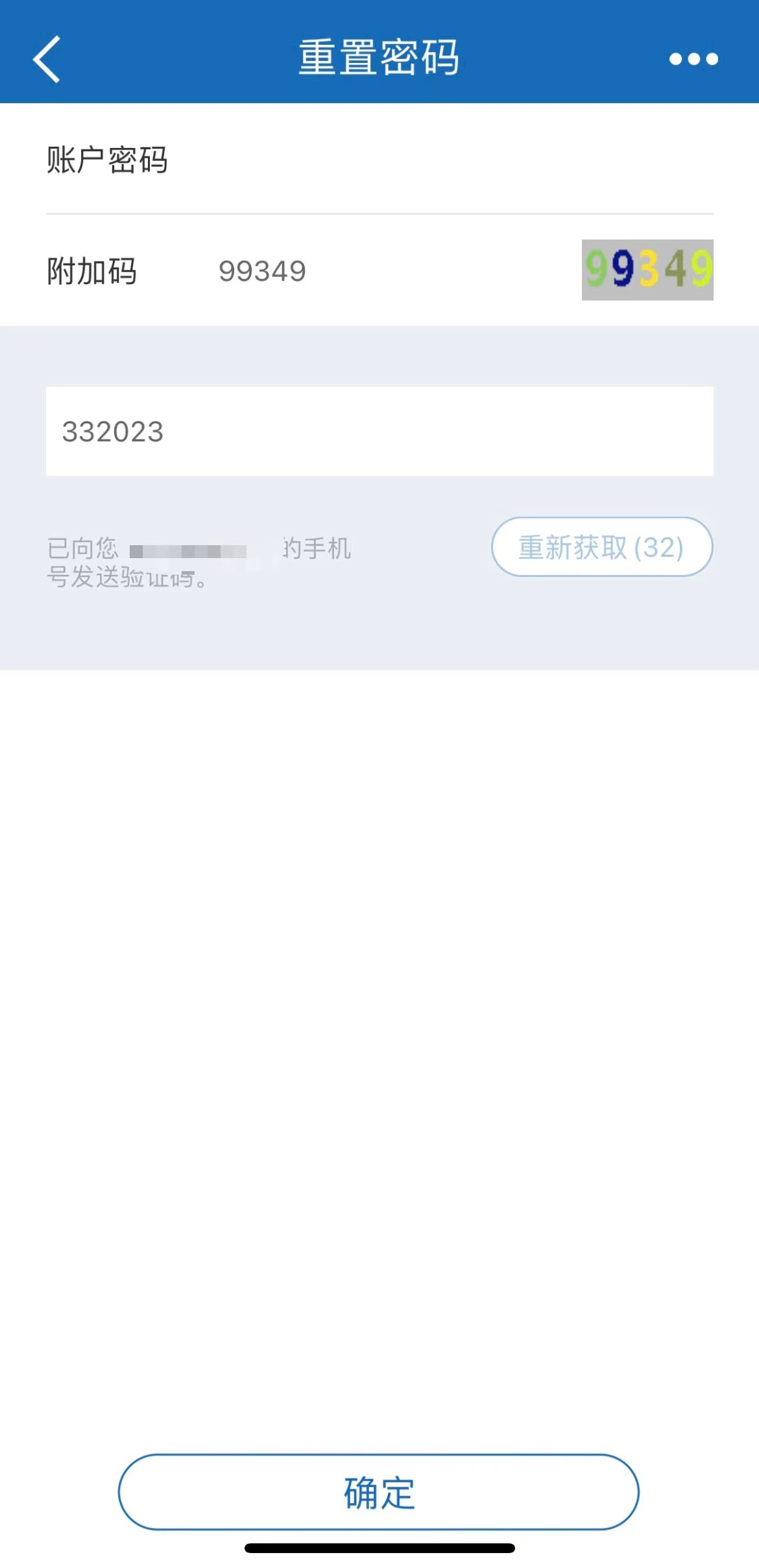﻿ 基于Python实现原生的登录验证码详情_python_脚本之家
python# 基于Python实现原生的登录验证码详情## 1、概述

`html `语法中，嵌入一张图片一般用 `img `标签实现，而 `img `标签对应的 `src `一般有以下几种写法

• 图片的本地路径
• 图片的`url`
• 图片的二进制数据（`base64`编码）

## 2、验证码实现的演进过程

### 2.1 路由及页面

```urlpatterns = [
......
# 图片验证码
url(r'^get_code/', views.get_code, name='gc'),
...
]

```

```...
<div class="form-group">
<label for="">验证码</label>
<div class="row">
<div class="col-md-6">
<input type="text" name="code" id="id_code" class="form-control">
</div>
<div class="col-md-6">
<img src="/get_code/" alt="" width="430" height="35" id="id_img">
</div>
</div>

</div>
<input type="button" class="btn btn-success" value="登陆" id="id_commit">
...
```

### 2.2 视图函数中验证码的推导

#### 2.2.1 图片发送到前端

```def get_code(request):
# 直接获取后端现成的图片二进制数据发送给前端
with open(r'static/img/test.jpg','rb') as f:
return HttpResponse(data)

```

#### 2.2.2 引入动态图片

```pip3 install pillow

```

• `Image`：生成图片
• `ImageDraw`：在图片上定义内容
• `ImageFont`：控制字体样式

```import random
from PIL import Image, ImageDraw, ImageFont

def get_random():
return random.randint(0, 255), random.randint(0, 255), random.randint(0, 255)

def get_code(request):
# 利用pillow模块动态产生图片
# img_obj = Image.new('RGB',(430,35),'green')  # RGB组合、图片尺寸、颜色
img_obj = Image.new('RGB',(430,35),get_random())  # 通过色值指定颜色
# 先将图片对象保存起来
with open('xxx.png','wb') as f:
img_obj.save(f,'png')
# 再将图片对象读取出来
with open('xxx.png','rb') as f:
return HttpResponse(data)

```

#### 2.2.3 内存管理模块图片

• `BytesIO`:临时存储数据，返回的时候数据是二进制
• `StringIO`:临时存储数据，返回的时候数据是字符串

```import random
from PIL import Image, ImageDraw, ImageFont

def get_random():
return random.randint(0, 255), random.randint(0, 255), random.randint(0, 255)

def get_code(request):
img_obj = Image.new('RGB', (430, 35), get_random())
io_obj = BytesIO()  # 生成一个内存管理器对象  你可以看成是文件句柄
img_obj.save(io_obj,'png')
return HttpResponse(io_obj.getvalue())  # 从内存管理器中读取二进制的图片数据返回给前端
```

#### 2.2.4 完整图片验证码

```import random
from PIL import Image, ImageDraw, ImageFont

def get_random():
return random.randint(0, 255), random.randint(0, 255), random.randint(0, 255)

def get_code(request):
# 写图片验证码
img_obj = Image.new('RGB', (430, 35), get_random())
img_draw = ImageDraw.Draw(img_obj)  # 产生一个画笔对象
# 引入本地的字体文件，指定字体样式及字体大小
img_font = ImageFont.truetype('static/font/222.ttf', 30)
# 随机验证码 五位数的随机验证码  数字 小写字母 大写字母
code = ''
for i in range(5):
random_upper = chr(random.randint(65, 90))  # 随机大写字母
random_lower = chr(random.randint(97, 122))  # 随机小写字母
random_int = str(random.randint(0, 9))  # 随机数字
# 每次从上面三个里面随机选择一个
tmp = random.choice([random_lower, random_upper, random_int])
# 将产生的随机字符串写入到图片上，需要调整每个字体所在的坐标变换
img_draw.text((i * 60 + 60, -2), tmp, get_random(), img_font)
# 拼接随机字符串
code += tmp
print(code)
# 随机验证码在登陆的视图函数里面需要要比对,所以要找地方存起来并且其他视图函数也能拿到
request.session['code'] = code
io_obj = BytesIO()
img_obj.save(io_obj, 'png')
return HttpResponse(io_obj.getvalue())

```

### 2.3 登录验证中使用验证码

```......
if request.method == 'POST':
back_dic = {'code': 1000, 'msg': ''}
code = request.POST.get('code')
# 1 先校验验证码是否正确  自己决定是否忽略大小写 统一转大写或小写再比较
if request.session.get('code').upper() == code.upper():
# 校验用户名和密码是否正确
if user_obj:
# 保存用户状态
back_dic['url'] = '/home/'
else:
back_dic['code'] = 2000
back_dic['msg'] = '用户名或密码错误'
else:
back_dic['code'] = 3000
back_dic['msg'] = '验证码错误'
return JsonResponse(back_dic)

```

### 2.4 前端页面点击自动刷新

```...
<div class="form-group">
<label for="">验证码</label>

<div class="row">
<div class="col-md-6">
<input type="text" name="code" id="id_code" class="form-control">
</div>
<div class="col-md-6">
<img src="/get_code/" alt="" width="430" height="35" id="id_img">
</div>
</div>

</div>
<input type="button" class="btn btn-success" value="登陆" id="id_commit">
...
<script>
\$("#id_img").click(function () {
// 获取标签之前的src
let oldVal = \$(this).attr('src');
\$(this).attr('src',oldVal += '?')
})
</script>

```

## 3、效果展示## 4、小结到此这篇关于基于Python实现原生的登录验证码详情的文章就介绍到这了,更多相关基于Python实现原生的登录验证码内容请搜索脚本之家以前的文章或继续浏览下面的相关文章希望大家以后多多支持脚本之家！